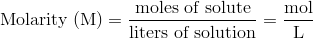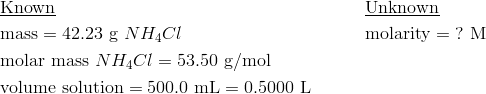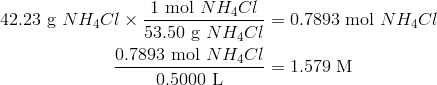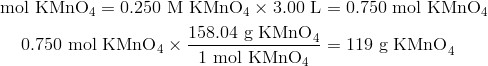## Molarity

### Learning Objectives

• Define molarity.
• Perform calculations involving molarity.

### How many molecules can be found in a reaction?

Chemists deal with amounts of molecules every day. Our reactions are described as so many molecules of compound A reacting with so many molecules of compound B to form so many molecules of compound C. When we determine how much reagent to use, we need to know the number of molecules in a given volume of the reagent. Percent solutions only tell us the number of grams, not molecules. A 100 mL solution of 2% NaCl will have a very different number of molecules than a 2% solution of CsCl. So we need another way to talk about numbers of molecules.

## Molarity

Chemists primarily need the concentration of solutions to be expressed in a way that accounts for the number of particles that react according to a particular chemical equation. Since percentage measurements are based on either mass or volume, they are generally not useful for chemical reactions. A concentration unit based on moles is preferable. The molarity (M) of a solution is the number of moles of solute dissolved in one liter of solution. To calculate the molarity of a solution, you divide the moles of solute by the volume of the solution expressed in liters.Note that the volume is in liters of solution and not liters of solvent. When a molarity is reported, the unit is the symbol M and is read as “molar”. For example a solution labeled as 1.5 M NH 3 is read as “1.5 molar ammonia solution”.

### Sample Problem: Calculating Molarity

A solution is prepared by dissolving 42.23 g of NH 4 Cl into enough water to make 500.0 mL of solution. Calculate its molarity.

Step 1: List the known quantities and plan the problem.The mass of the ammonium chloride is first converted to moles. Then the molarity is calculated by dividing by liters. Note the given volume has been converted to liters.

Step 2: Solve.The molarity is 1.579 M, meaning that a liter of the solution would contain 1.579 mol NH 4 Cl. Four significant figures are appropriate.

In a laboratory situation, a chemist must frequently prepare a given volume of solutions of a known molarity. The task is to calculate the mass of the solute that is necessary. The molarity equation can be rearranged to solve for moles, which can then be converted to grams. See sample problem 16.3.

### Sample Problem:

A chemist needs to prepare 3.00 L of a 0.250 M solution of potassium permanganate (KMnO 4 ). What mass of KMnO 4 does she need to make the solution?

Step 1: List the known quantities and plan the problem.

Known

• molarity = 0.250 M
• volume = 3.00 L
• molar mass KMnO 4 = 158.04 g/mol

Unknown

• mass KMnO 4 = ? g

Moles of solute is calculated by multiplying molarity by liters. Then, moles is converted to grams.

Step 2: Solve.When 119 g of potassium permanganate is dissolved into water to make 3.00 L of solution, the molarity is 0.250 M.

Watch a video of molarity calculations:

### Summary

• Calculations using the concept of molarity are described.

### Practice

Read the material and work the problems at the site below:

http://www.occc.edu/kmbailey/Chem1115Tutorials/Molarity.htm

### Review

1. What does M stand for?
2. What does molarity tell us that percent solution information does not tell us?
3. What do we need to know about a molecule in order to carry out molarity calculations?

## Glossary

• molarity (M): The number of moles of solute dissolved in one liter of solution.